﻿ Related Fixed Point Theorem for Mappings on Three Metric Spaces

### Related Fixed Point Theorem for Mappings on Three Metric Spaces

L. Bishwakumar, Yumnam RohenOPEN ACCESSPEER-REVIEWED

## Related Fixed Point Theorem for Mappings on Three Metric Spaces

L. Bishwakumar1, Yumnam Rohen2,1Modern College, Imphal, Pin-795005, India

2National Institute of Technology Manipur, Imphal-795001, India

### Abstract

In this note we obtained a new related fixed point theorem on three metric spaces of which one is compact. Here we consider three mappings, not all of which are necessarily continuous. Our result generalizes some earlier results.

• Bishwakumar, L., and Yumnam Rohen. "Related Fixed Point Theorem for Mappings on Three Metric Spaces." American Journal of Applied Mathematics and Statistics 2.4 (2014): 244-245.
• Bishwakumar, L. , & Rohen, Y. (2014). Related Fixed Point Theorem for Mappings on Three Metric Spaces. American Journal of Applied Mathematics and Statistics, 2(4), 244-245.
• Bishwakumar, L., and Yumnam Rohen. "Related Fixed Point Theorem for Mappings on Three Metric Spaces." American Journal of Applied Mathematics and Statistics 2, no. 4 (2014): 244-245.

 Import into BibTeX Import into EndNote Import into RefMan Import into RefWorks

### 1. Introduction

Related fixed point theorems on three metric spaces have been studied by several authors [1-8]. Fisher and Rao  proved a related fixed point theorem for three mappings on three metric spaces of which one is a compact metric space. The aim of this paper is to improve the result of Rao et. al.  and Fisher and Rao .

### 2. Main Results

We prove the following theorem.

2.1. Theorem. Let (X, d), (Y, ρ) and (Z, σ) be three metric spaces and T: XY, S:YZ and R:ZX be mappings satisfying the inequalities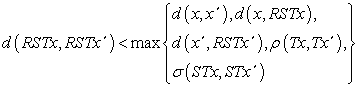(1)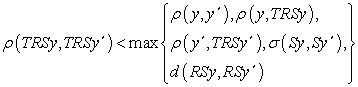(2)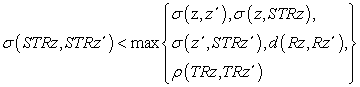(3)

for all x, x΄ in X , y, y΄ in Y and z, z΄ in Z. Further assume one of the following conditions:

(i) (X, d) is compact and RST is continuous.

(ii) (Y, ρ) is compact and TRS is continuous.

(iii) (Z, σ) is compact and STR is continuous.

Then RST has a unique fixed point w in X, TRS has a unique fixed point u in Y and STR has a unique fixed point v in Z. Further Su = v, Rv = w and Tw = u.

Proof: Suppose (i) holds. Define Φ(x) = d(x, RSTx) for x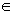X. Then there exists p in X such that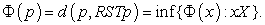Suppose that RSTRSTRSTpRSTRSTp.

Then STRSTRSTp≠STRSTp, TRSTRSTp≠TRSTp, RSTRSTp≠RSTp, STRSTp≠STp, TRSTp≠Tp, RSTp≠p.

Using (1) with x=RSTp and x΄=RSTRSTp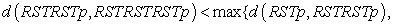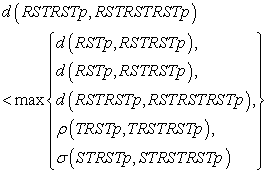so that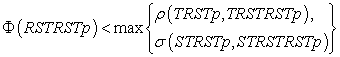(4)

Using (2) with y = Tp and y΄ = TRSTp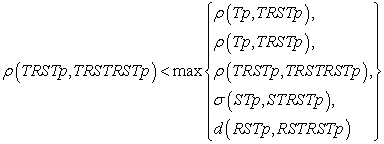so that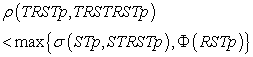(5)

Using (3) with z = STp and z΄ = STRSTp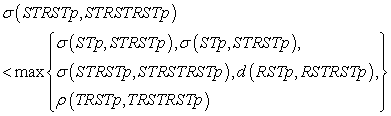so that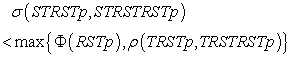(6)

From (4), (5) and (6) it follows that Φ(RSTRSTp) < Φ(RSTp), contradicting the existence of p.

Hence RSTRSTRSTp = RSTRSTp

Putting RSTRSTp = w in X, we have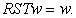Now let Tw = u in Y and Su = v in Z. Then Rv = RSu = RSTw = w and it follows that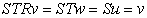and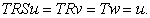To prove uniqueness, suppose RST has a second distinct fixed point w΄ in X.

Then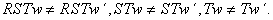Using (1) with x = w and x΄ = w΄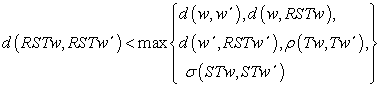so that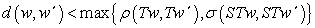(7)

Using (2) with y = Tw and y΄ = Tw΄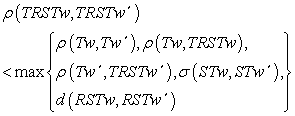so that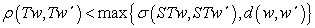(8)

Using (3) with z = STw and z΄ = STw΄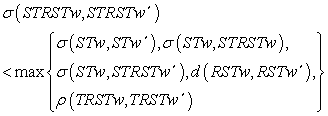so that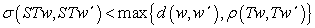(9)

From (7), (8) and (9), it follows that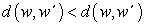so that w = w΄, proving the uniqueness of w.

Similarly we can show that v is the unique fixed point of STR and u is the unique fixed point of TRS.

It follows similarly that the theorem holds if (ii) or (iii) holds instead of (i).

Now we give the following example to illustrate our theorem.

Example. Let X = [0, 1], Y = (1, 2], Z = (2, 3] and let d = ρ = σ be the usual metric for the real numbers. Define T: XY, S: YZ and R: ZX by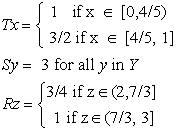Here Y and Z are not compact spaces and T and R not continuous. However all the conditions of theorem 2.1 are satisfied. Clearly,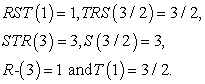### References

  Jain, R.K., Sahu, H.K. and Fisher, B. Related fixed point theorems for three metric spaces, Novi Sad J. Math. 26, 11-17, 1996.In article  Jain, R.K., Sahu, H.K. and Fisher, B. A related fixed point theorem on three metric spaces, Kyungpook Math. J. 36, 151-154, 1996.In article  Jain, R.K., Shrivastava, A.K. and Fisher, B. Fixed point theorems on three complete metric spaces, Novi Sad J. Math. 27, 27-35, 1997.In article  Jain, S. and Fisher, B. A related fixed point theorem for three metric spaces, Hacettepe J. Math. and Stat. 31, 19-24, 2002.In article  Nung, N.P. A fixed point theorem in three metric spaces, Math. Sem. Notes, Kobe Univ. 11, 77-79, 1983.In article  Rao, K.P.R., Srinivasa Rao, N. and Hari Prasad, B.V.S.N. Three fixed point results for three maps, J. Nat. Phy. Sci. 18, 41-48, 2004.In article  Rao, K.P.R., Hari Prasad, B.V.S.N. and Srinivasa Rao, N. Generalizations of some fixed point throrems in complete metric spaces, Acta Ciencia Indica, Vol. XXIX, M. No. 1, 31-34, 2003.In article  Rao, K.P.R. and Fisher, B. A related fixed point theorem on three metric spaces, Hacettepe J. Math. and Stat., Vol. 36(2), 143-146, 2007.In article